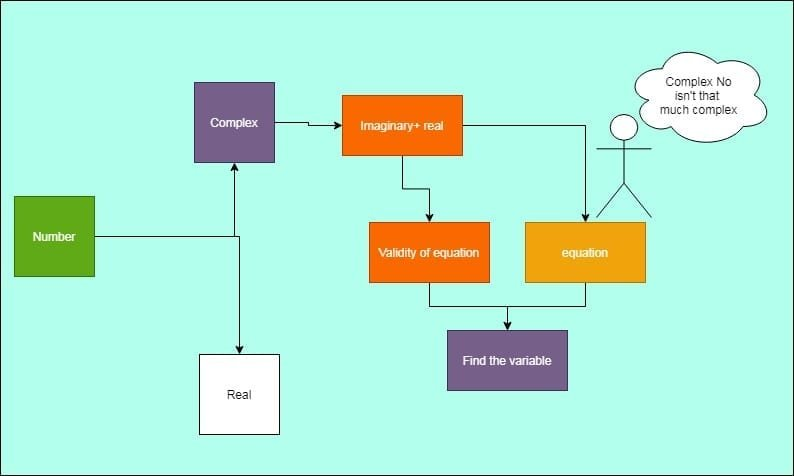How Cheenta works to ensure student success?
Explore the Back-Story

# Complex number- ISI entrance B. Stat. (Hons.) 2003- problem 5## Complex number

In complex number we have real and imaginary part mixed and $\sqrt{-1}$ is the basic unit and denoted by $i$. In the given question we have to find value of k for which the equation will be valid.

## Try the problem

How many integers $k$ are there for which $(1-i)^k=2^k$ ?

(A) One

(B) None

(C) Two

(D) More than one.

ISI entrance B. Stat. (Hons.) 2003 problem 5

Complex numbers

6 out of 10

Challenges and thrills of pre-college mathematics

## Use some hints

The complex number $(1-i)$ can be rationalized by multiplying numerator and denominator by $1+i$.

And we will get

$(1-i)=(1-i)\frac{(1+i)}{1+i}=\frac{2}{1+i}$

Now we will have $(\frac{2}{1+i})^k=2^k$

so, $(1+i)^k=1$, this is only possible when k=0,

So $k$ can have only one value, The option (A) is correct.

## Complex number

In complex number we have real and imaginary part mixed and $\sqrt{-1}$ is the basic unit and denoted by $i$. In the given question we have to find value of k for which the equation will be valid.

## Try the problem

How many integers $k$ are there for which $(1-i)^k=2^k$ ?

(A) One

(B) None

(C) Two

(D) More than one.

ISI entrance B. Stat. (Hons.) 2003 problem 5

Complex numbers

6 out of 10

Challenges and thrills of pre-college mathematics

## Use some hints

The complex number $(1-i)$ can be rationalized by multiplying numerator and denominator by $1+i$.

And we will get

$(1-i)=(1-i)\frac{(1+i)}{1+i}=\frac{2}{1+i}$

Now we will have $(\frac{2}{1+i})^k=2^k$

so, $(1+i)^k=1$, this is only possible when k=0,

So $k$ can have only one value, The option (A) is correct.

## Subscribe to Cheenta at Youtube

This site uses Akismet to reduce spam. Learn how your comment data is processed.

### Knowledge Partner